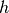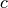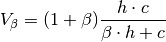# V-measure¶

The V-Measure is defined as the harmonic mean of homogeneityand completenessof the clustering. Both these measures can be expressed in terms of the mutual information and entropy measures of the information theory.Homogeneityis maximized when each cluster contains elements of as few different classes as possible. Completenessaims to put all elements of each class in single clusters.

References:

Andrew Rosenberg and Julia Hirschberg, 2007. “V-Measure: A conditional entropy-based external cluster evaluation measure”

The metric is implemented by the vmeasure function:

vmeasure(assign1, assign2; β = 1.0)

Compute V-measure value between two clustering assignments.

Parameters: assign1 – the vector of assignments for the first clustering. assign2 – the vector of assignments for the second clustering. β – the weight of harmonic mean of homogeneity and completeness. a V-measure value.
vmeasure(R, assign)

This method takes R, an instance of ClusteringResult, and the corresponding assignment vector assign as input, and computes V-measure value (see above).

vmeasure(R1, R2)

This method takes R1 and R2 (both are instances of ClusteringResult) and computes V-measure value (see above).

It is equivalent to vmeasure(assignments(R1), assignments(R1)).